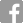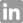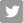×

# Quantitative Reasoning Section

## Analyze Data and Draw Conclusions

The Quantitative Reasoning section of the GMAT exam measures your ability to reason mathematically, solve quantitative problems, and interpret graphic data. It consists of 31 multiple-choice questions. You will have 62 minutes to complete it.

## Two Types of Questions in the Quantitative Section

There are two types of questions in the Quantitative Section – Problem Solving and Data Sufficiency. Both types of questions require some knowledge of arithmetic, elementary algebra and commonly known concepts of geometry. Rest assured that the difficulty of the questions stems from the logic and analytical skills required, not the underlying math skills. Note that you cannot use a calculator while working on the Quantitative section.

#### Problem Solving

• Measures your ability to use logic and analytical reasoning to solve quantitative problems.
• You solve the problem and indicate the best of five answer choices.

#### Data Sufficiency

• Measures your ability to analyze a quantitative problem, recognize which data is relevant, and determine at what point there are enough data to solve the problem.
• You will be given a problem that consists of a question and two statements. Using the data in the statements, plus your knowledge of math and everyday facts, you decide whether you have enough data in the statement to answer the question asked.

#### Sample Problem Solving Question

Directions

Solve the problem and indicate the best of the answer choices given.

Question

If u > t, r > q, s > t, and t > r, which of the following must be true?

1. u > s
2. s > q
3. u > r

(A) I only

(B) II only

(C) III only

(D) I and II

(E) II and III

#### Sample Data Sufficiency Question

Directions

This data sufficiency problem consists of a question and two statements, labeled (1) and (2), in which certain data are given. You have to decide whether the data given in the statements are sufficient for answering the question. Using the data given in the statements, plus your knowledge of mathematics and everyday facts (such as the number of days in July or the meaning of the word counterclockwise), you must indicate whether:

• Statement (1) ALONE is sufficient, but statement (2) alone is not sufficient to answer the question asked.
• Statement (2) ALONE is sufficient, but statement (1) alone is not sufficient to answer the question asked.
• BOTH statements (1) and (2) TOGETHER are sufficient to answer the question asked, but NEITHER statement ALONE is sufficient to answer the question asked.
• Statements (1) and (2) TOGETHER are NOT sufficient to answer the question asked, and additional data specific to the problem are needed.

Question

If a real estate agent received a commission of 6 percent of the selling price of a certain house, what was the selling price of the house?

(1) The selling price minus the real estate agent's commission was \$84,600.

(2) The selling price was 250 percent of the original purchase price of \$36,000.

(A) Statement (1) ALONE is sufficient, but statement (2) alone is not sufficient.

(B) Statement (2) ALONE is sufficient, but statement (1) alone is not sufficient.

(C) BOTH statements TOGETHER are sufficient, but NEITHER statement ALONE is sufficient.

(D) EACH statement ALONE is sufficient.

(E) Statements (1) and (2) TOGETHER are NOT sufficient.